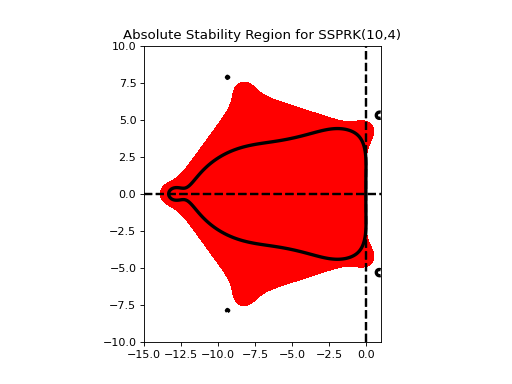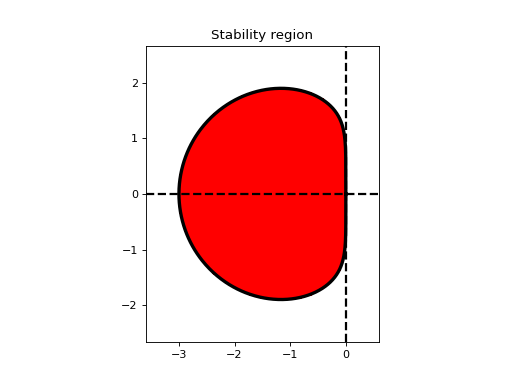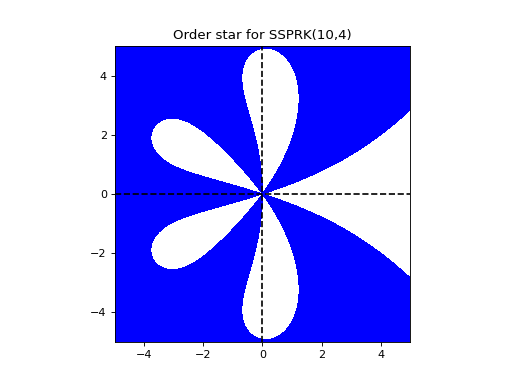# Analyzing Stability Properties¶

## Plotting the region of absolute stability¶

Region of absolute stability for the optimal SSP 10-stage, 4th order Runge-Kutta method:

from nodepy.runge_kutta_method import *
ssp104.plot_stability_region(bounds=[-15,1,-10,10])RungeKuttaMethod.plot_stability_region(N=200, color='r', filled=True, bounds=None, plotroots=False, alpha=1.0, scalefac=1.0, to_file=False, longtitle=True, fignum=None)[source]

The region of absolute stability of a Runge-Kutta method, is the set

$$\{ z \in C : |\phi (z)|\le 1 \}$$

where $$\phi(z)$$ is the stability function of the method.

Input: (all optional)
• N – Number of gridpoints to use in each direction
• bounds – limits of plotting region
• color – color to use for this plot
• filled – if true, stability region is filled in (solid); otherwise it is outlined
Example::
>>> from nodepy import rk
>>> rk4 = rk.loadRKM('RK44')
>>> rk4.plot_stability_region()
<matplotlib.figure.Figure object at 0x...>


Region of absolute stability for the 3-step Adams-Moulton method:

from nodepy.linear_multistep_method import *
am3.plot_stability_region()LinearMultistepMethod.plot_stability_region(N=100, bounds=None, color='r', filled=True, alpha=1.0, to_file=False, longtitle=False)[source]

The region of absolute stability of a linear multistep method is the set

$$\{ z \in C : \rho(\zeta) - z \sigma(zeta) \text{ satisfies the root condition} \}$$

where $$\rho(zeta)$$ and $$\sigma(zeta)$$ are the characteristic functions of the method.

Also plots the boundary locus, which is given by the set of points z:

$$\{z | z=\rho(\exp(i\theta))/\sigma(\exp(i\theta)), 0\le \theta \le 2*\pi \}$$

Here $$\rho$$ and $$\sigma$$ are the characteristic polynomials of the method.

References:
[leveque2007] section 7.6.1
Input: (all optional)
• N – Number of gridpoints to use in each direction
• bounds – limits of plotting region
• color – color to use for this plot
• filled – if true, stability region is filled in (solid); otherwise it is outlined

## Plotting the order star¶

Order star for the optimal SSP 10-stage, 4th order Runge-Kutta method:

from nodepy.runge_kutta_method import *
ssp104.plot_order_star()RungeKuttaMethod.plot_stability_region(N=200, color='r', filled=True, bounds=None, plotroots=False, alpha=1.0, scalefac=1.0, to_file=False, longtitle=True, fignum=None)[source]

The region of absolute stability of a Runge-Kutta method, is the set

$$\{ z \in C : |\phi (z)|\le 1 \}$$

where $$\phi(z)$$ is the stability function of the method.

Input: (all optional)
• N – Number of gridpoints to use in each direction
• bounds – limits of plotting region
• color – color to use for this plot
• filled – if true, stability region is filled in (solid); otherwise it is outlined
Example::
>>> from nodepy import rk
>>> rk4 = rk.loadRKM('RK44')
>>> rk4.plot_stability_region()
<matplotlib.figure.Figure object at 0x...>